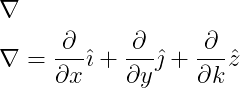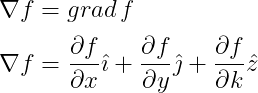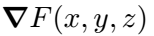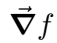# How to write a gradient operator(∇) in LaTeX?

In this tutorial, we will discuss how you can use the gradient operator in latex documents.

Scientifically, the gradient operator is denoted by the nabla(∇) symbol. And the gradient is always written in the form of a partial derivative.

\documentclass{article}
\begin{document}
$$\nabla$$
$$\nabla =\frac{\partial }{\partial x}\hat{\imath} + \frac{\partial }{\partial y}\hat{\jmath} +\frac{\partial }{\partial k}\hat{z}$$
\end{document}

Output :You need to use \imath and \jmath instead of unit vectors i and j. As a result, there will be no dots on the heads of i and j and that would look nice with the z unit vector.
Suppose, if the function is f. Then will be the gradient of the function

\documentclass{article}
\begin{document}
$$\nabla f = grad \: \textit{f}$$
\nabla f=\frac{\partial f}{\partial x}\hat{\imath} + \frac{\partial f}{\partial y}\hat{\jmath} +\frac{\partial f}{\partial k}\hat{z} $$\end{document} Output :You can easily represent the above tasks with the help of the physics package. And the best practice is to use a physics package for gradient operators. ## Use physics package for gradient operator in LaTeX The gradient command is used to define the gradient operator in physics packages. And the \gradient command is represented by \grad in short form. \documentclass{article} \usepackage{physics} \begin{document}$$ \grad F(x,y,z)$$\end{document} Output :The gradient operator is a vector. That is, you can denote the nabla(∇) symbol with a vector arrow above the nabla(∇) symbol without making it bold. \documentclass{article} \usepackage[arrowdel]{physics} \begin{document}$$ \grad f
\end{document}

Output :As you can see in the program above, arrowdel has been used as an optional argument with the physics package for vector arrow.#### Md Jidan Mondal

LaTeX expert with over 10 years of experience in document preparation and typesetting. Specializes in creating professional documents, reports, and presentations using LaTeX.